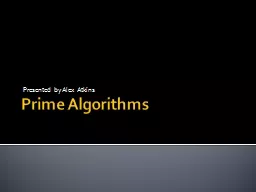# Prime Algorithms2016-06-22 42K 42 0 0

## Prime Algorithms - Description

Presented by Alex Atkins. What’s a Prime?. An integer p >= 2 is a prime if its only positive integer divisors are 1 and p. . Euclid proved that there are infinitely many primes. . The primary role of primes in number theory is stated in the Fundamental Theory of Arithmetic, which states that e.... ID: 372887 Download Presentation

Embed code:

## Prime Algorithms

Download Presentation - The PPT/PDF document "Prime Algorithms" is the property of its rightful owner. Permission is granted to download and print the materials on this web site for personal, non-commercial use only, and to display it on your personal computer provided you do not modify the materials and that you retain all copyright notices contained in the materials. By downloading content from our website, you accept the terms of this agreement.

### Presentations text content in Prime Algorithms

Slide1

Prime Algorithms

Presented by Alex Atkins

Slide2

What’s a Prime?

An integer p >= 2 is a prime if its only positive integer divisors are 1 and p. Euclid proved that there are infinitely many primes. The primary role of primes in number theory is stated in the Fundamental Theory of Arithmetic, which states that every integer n >= 2 is either a prime or can be expressed as a product of a primes.

Slide3

Prime Number Theorem

The Prime Number Theorem describes the asymptotic distribution of primes among positive integers.

The theorem states that a random integer between zero and some integer n, the probability the integer is a prime number is approximately 1/

ln

(n).

Slide4

Prime Number Theorem (2)

Asymptotic law of distribution of prime numbers: Pi(x) represents the prime-counting function, which denotes the number of primes less than or equal to x, for some real number x.x/Ln(x) approximates pi(x). The approximation produces a relative error that approaches zero as x approaches infinity.

Slide5

Trial Division

The simplest method of verifying

primality

is trial division.

The test is to determine whether n is a multiple of any integer between 2 and

sqrt

(n).

In an algorithm, the time can be improved by excluding even integers n >2 from being tested.

Slide6

What’s the Problem?

Inefficient & Slow

Primes are infinite and according to the prime number theorem, the probability that a number is prime becomes lower as our number n gets larger.

The larger the prime, the harder it is to find.

Slide7

Mersenne Primes

A Mersenne Prime is a prime number that is one less than a power of two.The largest prime numbers found are Mersenne Primes.47 Mersenne Primes have been found. The largest prime is (2^(43,112,609) – 1), and has over 12 million digits.

Slide8

Modern Primality Tests

Probabilistic vs. Deterministic

Probabilistic algorithms test if n is prime, by determining if n is composite or “probably prime”.

Deterministic algorithm will always produce a prime number given a particular input, using an underlying mathematical function.

Typically Probabilistic tests are done first, because they are quicker, but less robust.

Slide9

A few tests

Fermat

Primality

test

Probabilistic

O(k*log^(2+E)(n))

AKS

primality

test

Deterministic

O(log^(6+E)(n))

Slide10

Fermat’s Little Theorem

Theorem: If p is a prime, then the integer (a^p –a) is a multiple of p. Formula:

Slide11

AKS Algorithm

AKS

primality

test is unique.

Only

priamlity

test that posses all four properties:

General – checks any general number

Polynomial – Max run-time of algorithm

Deterministic – deterministically distinguishes between prime and composite numbers.

Unconditional – Does not depend on an unproven hypothesis.

Slide12

AKS Algorithm

The AKS algorithm is based on the theorem that an integer n is prime

iff

the polynomial congruence relation (1) holds for all integers a relatively prime to n.

(x – a)^n == (

x^n

– a ) (mod n)

(x – a)^n == (

x^n

–a ) (mod (

n,x^r

– 1))

(x –a )^n – (

x^n

–a) =

nf

+ (

x^r

– 1)g

Slide13

Slide14

Slide15

Slide16

Slide17

Slide18

Slide19

Slide20

Slide21